# Functions

## Overview

A function is a block of code that is run whenever the function is called within a program.

Functions are declared using the `function` keyword. For example, we create a function named`doSomething()`by typing:

``````function doSomething() {

// the code to do something

}``````

The curly braces {…} mark the beginning and end of the function's code block, which is the code run when the function is called. While computers are excellent at spotting the curly braces in a program, sometimes human eyes miss them. That's why it is standard practice to also indent the code in a block, making the code block stand out visually from the code around it.

Functions enable you to reuse your code instead of re-writing it every time, and they also make your programs easier to read and maintain.

## Define and Call a Function

In this example, we create a function named `drawSandwich()` that draws a sandwich. Another standard practice is to give functions descriptive names, so we know what they do, and to write them using camel case, capitalizing each word after the first one.

We start by declaring and defining the function:

``````function drawSandwich() {
context.save();
context.fillStyle = '#F5DEB3';
context.fillRect(20, 20, 200, 30);
context.fillRect(20, 100, 200, 30);
context.fillStyle = '#663300';
context.fillRect(30, 50, 180, 50);
context.fillStyle = '#FFD700';
context.fillRect(20, 62, 200, 8);
context.fillRect(20, 80, 200, 8);
context.restore();
}``````

Think of a function definition as a recipe. If we put a recipe for a sandwich up on the refrigerator, it doesn't mean there will be a sandwich waiting for us when we get home. We have to tell someone to use the recipe to make us a sandwich.

Right now, we have given the computer a recipe to follow if we ask it to draw a sandwich, but we haven't told it to draw a sandwich yet. To do that, we have to call the function using:

``drawSandwich();``

If we step through our program line by line, here is what happens:

First, the computer gets a reference to the canvas and stores it in the global variable `canvas`.

``var canvas = document.getElementById('functions_example1');``

Second, the computer gets a reference to the canvas's context and stores it in the global variable `context`.

``var context = canvas.getContext('2d');``

Then, the computer looks up and runs our function.

``drawSandwich();``

The computer recognizes that `drawSandwich()` is a function because of the parentheses (), so it searches for the function's definition in the program. It doesn't actually matter where we place the definition in the program; the computer will find it and run the function's code block.

In functions that use the context, it's generally a good idea to save and restore the context at the start and end of the function, respectively.

Quick Reference:

Message Log

## Challenge 1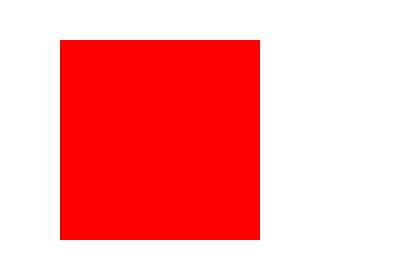What your drawing should look like

Define a function named `drawRedSquare()`. Inside the function's code block, set the context's fillStyle to `'#FF0000'` and use the `context.fillRect()` method to draw a filled rectangle at (60, 40) with a width of 200 and a height of 200.

Then, call the function `drawRedSquare()`.

The `context.fillRect()` method is simply a function that other programmers have created for us to use. The computer knows where to find its function definition, and it's called a method because it is a property of the context.

Message Log
##### Canvas (your drawing will display here)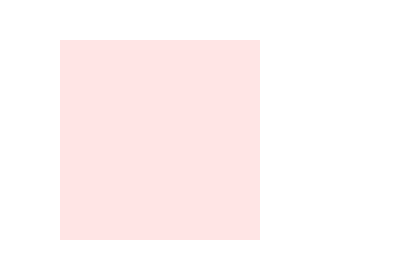## Pass Values into a Function

While our function `drawSandwich()` works, it's not very useful. It is hardcoded to always draw a sandwich with its top left corner at (20, 20). One of the reasons why we place code in functions is so we can use it over and over again without having to re-write it. But calling `drawSandwich()` more than once will only draw another sandwich directly on top of the first one.

To make the function more useful, we are going to re-define it to draw a sandwich at a set of coordinates we pass it, not just (20, 20). Values passed into a function are called arguments, and they are stored in local variables called parameters.

We add parameters to a function by listing them in the parentheses next to the function's name in its definition:

``````function drawSandwich(x, y) {

// code block

}``````

In this case, parameter `x` will store the value of the sandwich's x-coordinate and parameter `y` will store the value of the y-coordinate.

We then update the code inside the function to draw the rectangles for the bread, meat, and cheese at coordinates based on `x` and `y`:

``````function drawSandwich(x, y) {
context.save();
context.fillStyle = '#F5DEB3';
context.fillRect(x, y, 200, 30);
context.fillRect(x, y + 80, 200, 30);
context.fillStyle = '#663300';
context.fillRect(x + 10, y + 30, 180, 50);
context.fillStyle = '#FFD700';
context.fillRect(x, y + 42, 200, 8);
context.fillRect(x, y + 60, 200, 8);
context.restore();
}``````

There is a much easier way to draw a sandwich at coordinates (x, y) using the `context.translate()` method, but we will discuss that in the translate() lesson.

Now we can draw a stack of sandwiches by drawing three sandwiches at three different sets of coordinates:

``````drawSandwich(125, 20);
drawSandwich(20, 130);
drawSandwich(230, 130);``````

When we call the function `drawSandwich()` and pass it the arguments 125 and 20, the value 125 is stored in the parameter `x` and the value 20 in the parameter `y`.

To learn more about the coordinate system and variables, visit the Coordinates and Variables lessons.

Quick Reference:

Message Log

## Challenge 2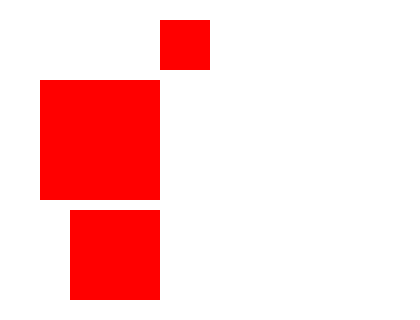What your drawing should look like

Re-define the function `drawRedSquare()` with the parameters `x`, `y`, and `s`, where `x` is the x-coordinate of the square, `y` is the y-coordinate, and `s` is the side length.

Then, use the function to draw three squares: the first at (160, 20) with a side length of 50; the second at (40, 80) with a side length of 120; and the third at (70, 210) with a side length of 90.

Message Log
##### Canvas (your drawing will display here)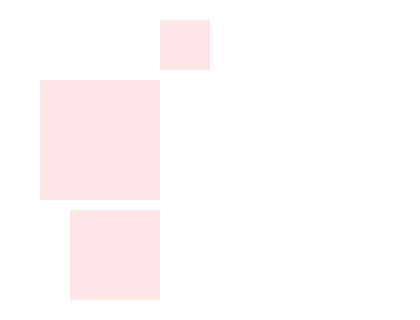## Challenge 3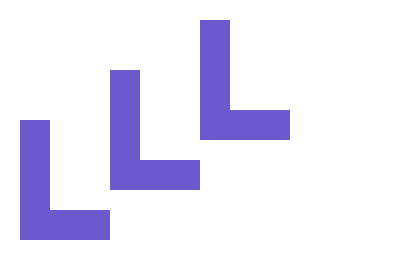What your drawing should look like

The function `drawTheLetterL()` is currently hardcoded to draw a letter L at (100, 50).

Re-define the function using parameters so it will draw a letter L at any set of coordinates passed into it.

Then, draw three letter L's: the first at (20, 120), the second at (110, 70), and the third at (200, 20);

Message Log
##### Canvas (your drawing will display here)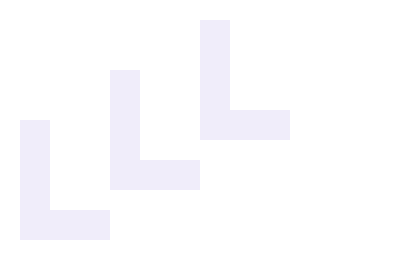## Return Values from Functions

One way to pass values into a function is through the use of parameters. One way to get values out of a function is through the use of return values.

In this example, we define the `calculateArea()` function, which calculates the area of a rectangle using the values stored in parameters `w` and `h`, and stores it in the local variable `area`. Because `area` is a local variable, it can't be accessed from outside of the function and it will no longer exist once the function ends. To get the value of `area` out of the function, we use a return statement.

``````function calculateArea(w, h) {
var area = w * h;
return area;
}``````

A return statement inside a function does two things. First, it ends the function even if there is more code to run below it. Second, it can return a value if one is provided. In this case, the `calculateArea()` function returns the value of `area`.

To use the `calculateArea()` function, we call it and store its return value in the global variable `area`. We can name two variables `area` in this situation because one is global and the other is local to the function `calculateArea()`. To learn more about variables and scope, visit the variables lesson.

``var area = calculateArea(200, 100);``

Finally, we draw the value stored in the global variable `area` on the canvas using the `context.fillText()` method.

``````context.font = '16px Arial';
context.fillStyle = 'Black';
context.fillText('The area is ' + area + '.', 10, 40);``````

Change the values passed into the `calculateArea()` function to see what happens. The function should automatically re-calculate the area drawn on the canvas.

To learn more about variables, visit the Variables lesson.

Quick Reference:

Message Log

## Challenge 4

Define a function named `randomIntegerFrom0To255()` to calculate and return a random integer from 0 to 255.

The `Math` object has a set of functions that other programmers have defined for us to use. Here is a full list of available

To calculate a random integer from 0 to 255, we will use the `Math.random()` function, which returns a random number from 0 to 1, but not including 1. By multiplying the value returned by the `Math.random()` function by 256, we will have a random number from 0 to 256, but not including 256. Then, to round that value down to an integer, we pass that value into the `Math.floor()` function. We want to round down because we don't want to round up to 256.

Here is what the code for the calculation looks like on one line:

``var i = Math.floor( 256 * Math.random() );``

The random integer from 0 to 255 is stored in the local variable `i`, which is what we want to return at the end of the function.

Call the `randomIntegerFrom0To255()` function and store its return value in a global variable. Then, draw the value stored in this global variable on the canvas at (10, 40) by passing it into the `context.fillText()` method. Every time you run the program, a new random integer from 0 to 255 should be drawn. If your program seems to be working, mark the challenge as complete by selecting "Yes, it looks good".

To learn more about rounding and generating random numbers, visit the round() / floor() / ceil() and random() lesson.

Quick Reference:

Message Log

## Call Functions from Inside of Other Functions

So far, we have been defining a function and calling it from the global scope (outside of any function). But it is possible to call one function from inside of another function.

In this example, we define four separate functions. The `drawRectangleWithArea()` function has four parameters that it uses to draw a green rectangle. It then calls the `calculateMidPoint()` function twice to calculate the coordinates for the center of the rectangle, storing those values in the local variables `midX` and `midY`. Finally, it passes the values of `midX`, `midY`, `w`, and `h` into the `printArea()` function.

``````function drawRectangleWithArea(x, y, w, h) {
context.save();
context.fillStyle = 'MediumSeaGreen';
context.fillRect(x, y, w, h);

var midX = calculateMidPoint(x, x + w);
var midY = calculateMidPoint(y, y + h);

printArea(midX, midY, w, h);

context.restore();
}``````

The `calculateMidPoint()` function has two parameters, `a` and `b`. It calculates the average of `a` and `b`, and returns the value using a return statement. Note that we aren't storing the average in a local variable and then returning the value of that variable. We are just returning the value directly, cutting out an unneccesary step.

``````function calculateMidPoint(a, b) {
return (a + b) / 2;
}``````

The `printArea()` function has four parameters. It passes the value of `w` and `h` into the `calculateArea()` function and stores the return value in the local variable `area`. It then sets a few properties the context uses to format text and calls the `context.fillText()` method to draw a string of text with the value of `area` at the coordinates (`x`, `y`), which is the midpoint of the rectangle.

``````function printArea(x, y, w, h) {
var area = calculateArea(w, h);

context.save();
context.font = '16px Arial';
context.fillStyle = 'Black';
context.textAlign = 'center';
context.textBaseline = 'middle';
context.fillText('My area is ' + area, x, y);
context.restore();
}``````

The `calculateArea()` function calculates the area of a rectangle using the values stored in parameters `w` and `h`, and returns the value. Again, we are cutting out a step by returning the value of the area directly.

``````function calculateArea(w, h) {
return w * h;
}``````

At this point, we have defined four functions. All four functions have a global scope because their function definitions have been declared at the top level of the program, not inside of another function. And because each function has a global scope, we can access and call them from inside any other function. That's why we can call the `calculateMidPoint()` and `printArea()` functions from inside the `drawRectangleWithArea()` function, and we can call the `calculateArea()` function from inside the `printArea()` function.

Although we have defined our four functions, our program isn't doing anything. It's stored references to the canvas and the canvas's context in the global variables `canvas` and `context`, respectively, but that's it. We still need to call the `drawRectangleWithArea()` function:

``drawRectangleWithArea(60, 40, 100, 80);``

This draws a green rectangle and prints the area at its midpoint.

Change the position and dimensions of the rectangle being passed into the `drawRectangleWithArea()` function. The drawing will automatically update to draw the new rectangle and print its area at its midpoint.

Visit the relevant lessons to learn more about `context.save()`, `context.fillRect()`, `context.fillStyle`, variables, and the context's coordinate system.

Quick Reference:

Message Log

## Challenge 5

Copy the code you wrote for the `randomIntegerFrom0To255()` function in Challenge 4. You are going to use that function to draw random rectangles with random colors.

In the definition for the `randomColor()` function, declare three local variables named `r`, `g`, and `b`, and use them to store three random integers from 0 to 255. We will use those three values to return a text string describing a color in rgb() notation.

In the definition for the `drawRandomRectangle()` function, declare two local variables named `w` and `h`, and use them to store two random integers from 0 to 255. Store a random color in the `context.fillStyle` property. Then, use the `context.fillRect()` method to draw a rectangle at position (`x`, `y`) with a width of `w` and a height of `h`.

Finally, call the `drawRandomRectangle()` function to draw one or more random rectangles on the canvas. Every time you run the program, the dimensions and color of the rectangles should change. If your program seems to be working, mark the challenge as complete by selecting "Yes, it looks good".

Quick Reference:

Message Log

## Make Programs Easier to Read and Maintain

Functions are helpful because they make it possible for us to re-use a block of code instead of typing it each time we need it. However, there are other important reasons to use functions.

Take a look at the function definition we created to draw sandwiches:

``````function drawSandwich(x, y) {
context.save();
context.fillStyle = '#F5DEB3';
context.fillRect(x, y, 200, 30);
context.fillRect(x, y + 80, 200, 30);
context.fillStyle = '#663300';
context.fillRect(x + 10, y + 30, 180, 50);
context.fillStyle = '#FFD700';
context.fillRect(x, y + 42, 200, 8);
context.fillRect(x, y + 60, 200, 8);
context.restore();
}``````

It's difficult to know what the function is doing just by glancing at it. If this was someone else's function or a function we had defined a long time ago, we would have to go through it carefully line by line to figure it out.

Now take a look at the same function re-written by calling other functions:

``````function drawSandwich(x, y) {
context.save();

drawBread(x, y);
drawRoastBeef(x + 10, y + 30);
drawCheeseSlice(x, y + 42);
drawCheeseSlice(x, y + 60);
drawBread(x, y + 80);

context.restore();
}``````

The function is much easier to read and understand, and it is also much easier to maintain. For example, if we want to update our cheese slices, we can do it in one place in the program, instead of in multiple places scattered throughout the program.

Quick Reference:

Message Log

## Pass Functions into Other Functions

Besides calling functions from inside of other functions, we can also pass functions into other functions and store them in parameters.

Why would we want to do that? Well, when we define function `a()` to call function `b()`, we are hardcoding function `a()` to call function `b()`. Most of the time, that's what we want. But sometimes we want function `a()` to a call a function to be determined later.

In this example, we define the `drawPlate()` function, which has three parameters:

``````function drawPlate(x, y, drawFood) {
context.save();
context.fillStyle = '#FFFFFF';
context.strokeStyle = '#999999';
context.fillRect(x - 120, y - 20, 240, 20);
context.strokeRect(x - 120, y - 20, 240, 20);
context.restore();

if (drawFood === undefined) {
return;
}

drawFood(x, y - 20);
}``````

The parameters `x` and `y` are the coordinates of the bottom center point of the plate. We draw a white rectangle with a gray outline centered at `x` and sitting on top of `y`.

Then, we do something odd. We check if the parameter `drawFood` is `undefined`. If a variable is undefined, it means a value was never assigned to it. If that's the case here, we are going to end the function with a return statement. There are other ways we could have done this, but we wanted to show you how to end a function early.

If `drawFood` is not undefined, then we call it as a function. Notice the parentheses () after the parameter name. We are calling it as a function and passing it the coordinates of the top center point of the plate. This is where we want the food drawn on the plate to be positioned.

To draw a sandwich on top of a plate, we have refactored the `drawSandwich()` function so it now draws the sandwich centered at the parameter `x` and on top of `y`.

``````function drawSandwich(x, y) {
context.save();

drawBread(x - 100, y - 110);
drawRoastBeef(x - 90, y - 80);
drawCheeseSlice(x - 100, y - 68);
drawCheeseSlice(x - 100, y - 50);
drawBread(x -100, y - 30);

context.restore();
}``````

To draw a piece of cake on top of a plate, we have defined the `drawCake()` function:

``````function drawCake(x, y) {
context.save();
context.fillStyle = '#FFFF00';
context.fillRect(x - 90, y - 180, 180, 180);
context.fillStyle = '#CD853F';
context.fillRect(x - 90, y - 8, 180, 8);
context.fillRect(x - 90, y - 51, 180, 8);
context.fillRect(x - 90, y - 94, 180, 8);
context.fillRect(x - 90, y - 137, 180, 8);
context.fillRect(x - 90, y - 180, 180, 8);
context.fillRect(x - 90, y - 180, 8, 180);
context.restore();
}``````

Putting everything together, we draw one plate with a piece of cake and one plate with a sandwich by calling:

``````drawPlate(140, 240, drawCake);
drawPlate(400, 240, drawSandwich);``````

Note that we are passing the name of the `drawCake()` or `drawSandwich()` function without putting parentheses () after them. We use parentheses to call a function. Here we are treating the function name as a variable that we are passing into the `drawPlate()` function to be called later. If we don't pass in a function at all, the plate will be drawn empty.

When we call the `drawCake()` or `drawSandwich()` functions inside of the `drawPlate()` function after passing them into the function as arguments, they behave as local functions that have been declared inside of the `drawPlate()` function, not as global functions. This means they have access to the local variables declared inside of the `drawPlate()` function.

Quick Reference:

Message Log

## Anonymous Functions

In JavaScript, we can also define anonymous functions.

In this example, we will draw a purple square on a plate by passing the `drawPlate()` function an anonymous function.

``````drawPlate(140, 240, function(x, y) {
context.save();
context.fillStyle = 'Purple';
context.fillRect(x - 60, y - 120, 120, 120);
context.restore();
});``````

We could have defined a `drawPurpleSquare()` function and passed it into the `drawPlate()` function by name, but sometimes we don't want to bother naming a function we will only use once.

It is also possible to save an anonymous function in a variable to be called later:

``````var drawPurpleSquare = function(x, y) {
context.save();
context.fillStyle = 'Purple';
context.fillRect(x - 60, y - 120, 120, 120);
context.restore();
};``````

When a function is declared with a name, it is automatically stored in a variable with the same name. But in JavaScript, functions are actually objects, so we can store them and pass them like any other object.

Quick Reference:

Message Log

## Ready for the next lesson?

Next up, the "For Loops" lesson >